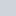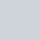### Implement a Plugin Architecture in Python with Only Two Lines of Code!, ,May 30, 2022 @ 12:58 pm, by raindog308I’ve been doing a ton of Python coding lately and one thing I’ve really appreciated is how easy it is to implement a plugin system.

When would you use this?  If you’re shipping code, you may want to include hooks for your end users to extend your code.  If you document a plugin interface, they can write their own custom code.

In my case, I’m using Python’s multiprocessing module to fire off a variety of jobs.  The core engine doesn’t change much but I’m writing all sorts of jobs.  A plugin architecture allows me to keep the engine static and do my work in the plugins.  It’s a very nice way to break up code (and although I won’t get into it here, using Python exception handling, you can make sure that if the plugin is broken it doesn’t blow up the rest of your code).

Plugins are so easy to use that I’ve been using them in all kinds of code.  Let me show you how they work.  All you really need is (1) the importlib module, and (2) a well-defined interface.

In this case, I’m going to implement a simple calculator.  Add, subtract, multiply, and divide will each by a plugin (so in theory we could extend it with more functions).  because this is an example, each plugin will just perform the requested operation on two numbers.  I’m also skipping all the error-checking and exception-handling (e.g., what if the someone calls the plugin with a string instead of an int, or only one argument, etc.)

I’ve created a directory called /lowendbox and under that, a directory called ‘plugins’.  In that directory, here is the ‘add.py’ plugin:

```#!/usr/bin/python3

class Plugin:

def calculate(self, *args):
return int(args) + int(args)```

Very simple, but there are two key points:

1. The Plugin class will be the same class defined in add.py, sub.py, multiply.py, and divide.py.  This will make sense shortly.
2. The calculate() method signature will be the same for each plugin.  This is how we actually execute the plugin.

The code for sub.py, etc. is identical except for the mathematical operation in calculate() so I won’t repeat each of them.

Now let’s look at calc.py, our calculator that will be using these plugins.

```#!/usr/bin/python3

plugin_dir = '/lowendbox/plugins'
import importlib, os, re, sys
sys.path.append(plugin_dir)

plugins = {}
for plugin_file in sorted(os.listdir(plugin_dir)):
if re.search('\.py\$', plugin_file):
module_name = re.sub('\.py\$', '', plugin_file)
module = importlib.import_module(module_name)
plugins[module_name] = module.Plugin()
print ("   %s" % ( module_name ))

print ("10 + 20 = %d" % plugins['add'].calculate(10,20) )
print ("18 - 11 = %d" % plugins['sub'].calculate(18, 11) )
print ("-3 * 6 = %d" % plugins['multiply'].calculate(-3, 6) )
print ("12 / 2 = %d" % plugins['divide'].calculate(12, 2) )```

Now, I said “implement in two lines” and that is clearly more than two lines.  I highly favor code that is more explicit over code that is more compact, but you can get the bolded parts down to two lines if you really must:

```for plugin_file in glob.glob("%s/*.py" % ( plugin_dir )):
plugins[ re.sub('\.py\$', '', os.path.basename(plugin_file)) ] =
importlib.import_module( re.sub('\.py\$', '',
os.path.basename(plugin_file))).Plugin()
```

I folded lines so they’d fit. But I think the the original is a lot easier to read! There may be a simpler way to shrink this – I’m an old Perl guy so I tend to use regexes for everything, but there’s also context (“with”) and filter().

Okay, okay, there’s also the “import importlib” statement and whatever you put in the Plugin file.  I use clickbait titles.  Sue me.

But I digress.

Here is the output:

```calculator starting.  loading plugins:
divide
multiply
sub

10 + 20 = 30
18 - 11 = 7
-3 * 6 = -18
12 / 2 = 6```

Let’s walk through the main points.

```plugins = {}
```

I’ve chosen to put the plugins in a dict of plugin name -> plugin module.

```for plugin_file in sorted(os.listdir(plugin_dir)):
if re.search('\.py\$', plugin_file):
module_name = re.sub('\.py\$', '', plugin_file)
module = importlib.import_module(module_name)
plugins[module_name] = module.Plugin()
print ("   %s" % ( module_name ))
```

This is the heart of the code. We look in the plugins directory and for each file, we use a regular expression (re.search) make sure it ends in “.py” (so this skips the pycache directory, old vim swap files, etc.)

For each .py file there (for example, ‘add.py’), we strip off the “.py” to get the module name. Then we import that module into a variable called “module”. In the next line, instantiate the Plugin() class from that module and put it in our plugins dict.

You could also do something like this:

```plugins[module_name] = importlib.import_module(module_name)

In this second example, you’re storing the module in the dict, whereas in my implementation, we’re storing the instantiated Plugin object in the dict. If you wanted to instantiate many instances of your plugin, you’d want to store the module.

Now let’s exercise it. For each operation, we use our dict to find the Plugin object, and then call its calculate() method.

```print ("10 + 20 = %d" % plugins['add'].calculate(10,20) )
print ("18 - 11 = %d" % plugins['sub'].calculate(18, 11) )
print ("-3 * 6 = %d" % plugins['multiply'].calculate(-3, 6) )
print ("12 / 2 = %d" % plugins['divide'].calculate(12, 2) )
```

So in just a couple lines of code, we have a full-functioned plugin system. Give it a try and see what this approach can do for your code!

1.•